Reach Us+44-1522-440391
Meta-Analysis of Test Accuracy Studies with Multiple and Missing Thresholds: A Multivariate-Normal Model | OMICS International
Journal of Biometrics & Biostatistics

Like us on:

All submissions of the EM system will be redirected to Online Manuscript Submission System. Authors are requested to submit articles directly to Online Manuscript Submission System of respective journal.

# Meta-Analysis of Test Accuracy Studies with Multiple and Missing Thresholds: A Multivariate-Normal Model

Richard D Riley1*, Yemisi Takwoingi1, Thomas Trikalinos2, Apratim Guha3, Atanu Biswas4, Joie Ensor1, R Katie Morris5,6 and Jonathan J Deeks1

1School of Health and Population Sciences, Public Health Building, University of Birmingham, Edgbaston, Birmingham, B15 2TT, UK

2Center for Evidence-based Medicine, Center for Statistical Sciences, and Department of Health Services, Policy & Practice, Brown University School of Public Health, Brown University, Providence, RI 02912, USA

3Production and Quantitative Methods Area, Indian Institute of Management, Ahmedabad-380015, India

4Applied Statistics Unit, Indian Statistical Institute, 203 B.T. Road, Kolkata–700 108, India

5Centre for Women's & Children Health and the School of Clinical and Experimental Medicine, College of Medical and Dental Sciences; University of Birmingham, Birmingham, B15 2TT, UK

6Fetal Medicine Centre, Birmingham Women's Hospital NHS Foundation Trust, Birmingham, UK

*Corresponding Author:
Richard D. Riley
School of Health and Population Sciences
Public Health Building, University of Birmingham
Edgbaston, Birmingham, B15 2TT, UK
Tel: 0121 414 7508
Fax: 0121 414 7878
E-mail: [email protected]

Received date: April 04, 2014; Accepted date: May 15, 2014; Published date: May 20, 2014

Citation: Riley RD, Takwoingi Y, Trikalinos T, Guha A, Biswas A, et al. (2014) Meta-Analysis of Test Accuracy Studies with Multiple and Missing Thresholds: A Multivariate-Normal Model. J Biomet Biostat 5:196. doi:10.4172/2155-6180.1000196

Copyright: © 2014 Riley RD, et al. This is an open-access article distributed under the terms of the Creative Commons Attribution License, which permits unrestricted use, distribution, and reproduction in any medium, provided the original author and source are are credited.

Visit for more related articles at Journal of Biometrics & Biostatistics

#### Abstract

Background: When meta-analysing studies examining the diagnostic/predictive accuracy of classifications based on a continuous test, each study may provide results for one or more thresholds, which can vary across studies. Researchers typically meta-analyse each threshold independently. We consider a multivariate meta-analysis to synthesise results for all thresholds simultaneously and account for their correlation.
Methods: We assume that the logit sensitivity and logit specificity estimates follow a multivariate-normal distribution within studies. We model the true logit sensitivity (logit specificity) as monotonically decreasing (increasing) functions of the continuous threshold. This produces a summary ROC curve, a summary estimate of sensitivity and specificity for each threshold, and reveals the heterogeneity in test accuracy across studies. Application is made to 13 studies of protein:creatinine ratio (PCR) for detecting significant proteinuria in pregnancy that each report up to nine thresholds, with 23 distinct thresholds across studies.
Results: In the example there were large within-study and between-study correlations, which were accounted for by the method. A cubic relationship on the logit scale was a better fit for the summary ROC curve than a linear or quadratic one. Between-study heterogeneity was substantial. Based on the summary ROC curve, a PCR value of 0.30 to 0.35 corresponded to maximal pair of summary sensitivity and specificity. Limitations of the proposed model include the need to posit parametric functions for the relationship of sensitivity and specificity with the threshold, to ensure correct ordering of summary threshold results, and the multivariate-normal approximation to the within-study sampling distribution. Conclusion: The joint analysis of test performance data reported over multiple thresholds is feasible. The proposed approach handles different sets of available thresholds per study, and produces a summary ROC curve and summary results for each threshold to inform decision-making.

#### Keywords

Multivariate meta-analysis; Test accuracy; Diagnostic test; Summary ROC curve; Correlation; Sensitivity; Specificity

#### Introduction

In the evaluation of diagnostic or predictive tests, meta-analysis methods are needed to synthesise the evidence about test accuracy from multiple studies. Most meta-analysis methods proposed in the literature consider a single two by two table from each study, which provides the number of true positives, true negatives, false positives, and false negatives [1-4]. However, when the test is measured on a continuous scale studies often report test performance at multiple thresholds, each relating to a different choice of threshold above which test results are classed as 'positive' and below which test results are classed as 'negative', either by reporting multiple two by two tables or labelled ROC curves. In this situation, researchers may select single thresholds per study, to allow estimation of a summary ROC curve using standard metaanalysis approaches [1-8]; or they may do a separate meta-analysis for each reported threshold .

However, a common problem is that most studies do not report the same set of thresholds. For example, in an evaluation of the spot protein:creatinine ratio (PCR) for detecting significant proteinuria in pregnancy, Morris et al. extracted tables for 23 different thresholds across 13 studies; eight of the thresholds were considered by just one study, but the other 15 thresholds were considered in two or more studies (Table 1), with a maximum of six studies for any threshold . In such situations, an approach that considers meta-analysis for each threshold independently will omit any studies that do not report the threshold of interest, and thus also ignore information from other thresholds that are available in those studies. In particular, test accuracy results from neighbouring thresholds are likely to be quite similar, and results for a missing threshold are bounded between any pair of higher and lower thresholds that are available. Statistically it is appealing to utilise such related information and, if possible, 'borrow strength' by considering all thresholds simultaneously [11-14].

First Author threshold ID, t threshold value, x TP FP FN TN Total High proteinuria Normal proteinuria
Al Ragib 1 0.13 35 51 4 95 185 39 146
6 0.18 33 42 6 104
7 0.19 33 39 6 107
8 0.2 31 38 8 108
22 0.49 29 23 10 123
Durnwald 3 0.15 156 35 12 17
8 0.2 152 27 16 25
15 0.3 136 23 32 29 220 168 52
19 0.39 123 14 45 38
20 0.4 120 12 48 40
23 0.5 106 9 62 43
Dwyer 3 0.15 54 28 2 32 116 56 60
5 0.17 51 25 5 35
7 0.19 50 18 6 42
12 0.24 41 8 15 52
14 0.28 37 3 19 57
19 0.39 31 0 25 60
Leonas 15 0.3 277 7 5 638 927 282 645
Ramos 23 0.5 25 1 1 20 47 26 21
Robert 15 0.3 27 4 2 38 71 29 42
Rodriguez 2 0.14 69 34 0 35 138 69 69
3 0.15 68 34 1 35
4 0.16 68 26 1 43
5 0.17 65 25 4 44
6 0.18 62 24 7 45
7 0.19 62 21 7 48
8 0.2 60 19 9 50
9 0.21 60 17 9 52
Saudan 8 0.2 14 27 0 59 100 14 86
13 0.25 13 14 1 72
15 0.3 13 7 1 79
18 0.35 12 4 2 82
20 0.4 11 3 3 83
21 0.45 10 0 4 86
Schubert 3 0.15 9 3 0 3 15 9 6
4 0.16 9 2 0 4
Shahbazian 8 0.2 35 2 3 41 81 38 43
Taherian 2 0.14 67 7 6 20 100 73 27
3 0.15 67 3 6 24
4 0.16 65 1 8 26
5 0.17 64 1 9 26
6 0.18 63 0 10 27
8 0.2 59 0 14 27
Wheeler 9 0.21 59 13 9 45 126 68 58
Yamasmit 7 0.19 29 6 0 7 42 29 13
9 0.21 29 5 0 8
10 0.22 29 4 0 9
11 0.23 28 3 1 10
12 0.24 28 2 1 11
13 0.25 28 1 1 12
14 0.28 27 1 2 12
16 0.31 26 1 3 12
17 0.32 25 1 4 12

Table 1: PCR results for each threshold in each of the 13 studies of Morris et al. .

In this article we propose a new approach for meta-analysing diagnostic test accuracy studies when there are multiple threshold results per study, when the studies use the same (or similarly validated or standardised) methods of measuring the continuous test (e.g. blood pressure or a continuous biomarker, like prostate-specific antigen or proteinuria). Hamza et al.  proposed a multivariate random-effect meta-analysis approach and applied it when all studies report all of the thresholds of interest. However, we consider approaches that allow different sets of available thresholds per study and can accommodate studies that only provide one threshold. The Hamza approach models the (linear) relationship between threshold value and test accuracy within each study, but this is not possible in studies that only report one threshold. The Hamza method is also prone to convergence problems, prompting Putter et al. to propose an alternative survival model framework for meta-analysing the multiple thresholds . However this too requires the multiple thresholds to be available in all studies. Others have also considered the multiple threshold issue [13,16-20]. Here we extend the bivariate-normal meta-analysis model proposed by Reitsma et al. , which originally only allowed one threshold result per study. We propose a model that accounts for within-study correlations in the sensitivities and specificities at various thresholds (induced because estimates for different thresholds are from the same patients), and allows for relationships between test performance metrics at the between-study level (induced because studies can use different sets of thresholds). The approach incorporates all reported thresholds, even those reported in just one study, and can include all available studies, even if they provide just one threshold. It leads to a summary ROC curve, and summary sensitivity and specificity results for each threshold.

The article is structured as follows. In section 2 we describe the motivating PCR dataset. In Section 3 we introduce the model and its possible specifications, describe the estimation process, obtain a summary ROC curve, and summarise test performance at each threshold. In Section 4 we apply the methods to the PCR data, and Section 5 concludes with some discussion.

#### Motivating Example: Identification of Significant Proteinuria in Patients with Suspected Pre-eclampsia

Pre-eclampsia is a major cause of maternal and perinatal morbidity and mortality, and occurs in 2-8% of all pregnancies [21-24]. The diagnosis of pre-eclampsia is determined by the presence of elevated blood pressure combined with significant proteinuria (≥ 0.3 g per 24 hour) after the 20th week of gestation in a previously normotensive, non-proteinuric patient . The gold-standard method for detection of significant proteinuria is the 24 hour urine collection, but this is cumbersome, time consuming and inconvenient, to patients as well as hospital staff. A rapid and accurate diagnostic test for significant proteinuria is needed for timely decision-making.

The spot PCR has been shown to be strongly correlated with 24 hour protein excretion, and thus is a potential diagnostic test for significant proteinuria. Morris et al.  performed a systematic review and meta-analysis to assess the diagnostic accuracy of PCR for the detection of significant proteinuria in patients with suspected pre-eclampsia. Thirteen relevant studies were identified, and in each study the reference standard was proteinuria greater than or equal to 300 mg in urine over 24 hours. Across the 13 studies, 23 different threshold values were considered for PCR, ranging from 0.13 to 0.50 (as this is a ratio, there are no units). Five studies provided diagnostic accuracy results (i.e. a 2 by 2 table showing the number of true positives, false positives, false negatives, and true negatives) for just one threshold, but the other eight studies reported results for each of multiple thresholds, up to a maximum of nine thresholds (Yamasmit study). Eight of the 23 thresholds were considered by just one study, but the other 15 thresholds were considered in two or more studies, up to a maximum of six studies (for threshold 0.20). The studies and thresholds are summarised in Table 1.

Meta-analysis is important here to summarise the diagnostic accuracy of PCR at each threshold from all the published evidence, to help ascertain whether PCR is a useful diagnostic test and to give insight into clinically useful thresholds.

#### Methods

To meta-analyse diagnostic test studies with multiple thresholds, we consider a multivariate meta-analysis model that extends the bivariate model proposed by Reitsma et al. . Multivariate normality of the logit sensitivity and logit specificity is assumed for the within-study sampling distribution, which is an approximation to the exact multinomial distribution . However, multivariate normal distributions with randomeffects are easier to fit than multinomial models and more readily available in standard software packages such as Stata . They enable the correlation between thresholds to be estimated and utilised. This is crucial as at the within-study level the same patients are contributing to results at each threshold and this causes the multiple logit sensitivity estimates to be correlated across thresholds, and similarly the multiple logit specificity estimates to be correlated. Across studies, there is also potential between-study correlation in the true logit sensitivity and true logit specificity values. This multivariate-normal framework utilises all these correlations in the meta-analysis , and enables the joint, rather than separate, synthesis of multiple thresholds. The approach is a two-step process, with the second stage producing the summary meta-analysis results for each threshold and a summary ROC curve, if desired.

Step 1: Estimating threshold results and their variance-covariance matrix in each study

Let there be i=1 to m studies that measure a continuous test result on nli diseased patients and n0i non-diseased patients, whose true disease status is provided by a reference standard. In each study, at a particular threshold value, x, each patient's measured test value is classed as either 'positive' (≥ x) or 'negative' (<x). Then summarising test results over all patients produces aggregate data in the form of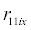, the number of truly diseased patients in study i with a positive test result at threshold x, and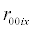, the number of non-diseased patients in study i with a negative test result. The observed sensitivity at threshold value of x in each study is thus simply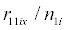and the observed specificity is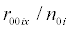; a logit transformation can then be applied to obtain logit sensitivity and logit specificity estimates at each threshold. If sensitivity or specificity is 0 or 1, then to enable logit estimates a continuity correction of 0.5 could be added to eachand, and 1 added to each nli and n0i. We focus here only on the logit transformation, as it is the most common one used in diagnostic test meta-analysis, but Chu et al. highlight that others (e.g. log-log or probit) can also be used .

To obtain the within-study variance-covariance matrix for the logit estimates, containing their variances and correlations, one can maximise the multinomial likelihood for each study, written as a function of the probabilities that test values fall between particular thresholds. The process is now described. First, in each study order the available thresholds. For example, in the Al Ragib study (Table 1) the threshold values of x=0.13, 0.18, 0.19, 0.2, and 0.49 could be considered ti=1, 2, 3, 4, and 5 respectively for this study, with max (ti)=5 (i.e. the total number of thresholds in study i). Then for the diseased people define probabilities, p1iSi, where Si=1 to max (ti) and: p1i1 is the probability a patient's test value falls below threshold 1, p1i2 is the probability a patient's test value falls between threshold 1 and 2, and so on, with the constraint that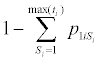is the probability a patient's test value falls above threshold max (ti). Similarly define max (ti) probabilities for non-diseased people, termed p0iSi. Then write the multinomial likelihood in terms of these probabilities for diseased patients, li(1), and non-diseased patients, li (0).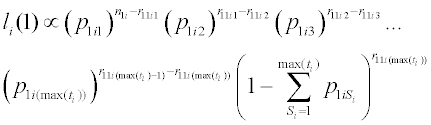(1)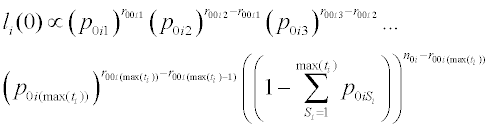(2)

and use statistical software (e.g. SAS Proc NLMIXED  or Stata 'maximize' ) to maximise the log likelihoods and subsequently obtain estimates of the logit sensitivity at threshold ti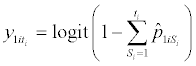(3)

and the logit specificity at threshold ti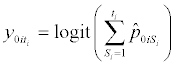(4)

and their variance-covariance matrix, for example using the delta method [31,32]. Example SAS code is available on request from the first author. For example, for the Al Ragib study (Table 1) the likelihoods are: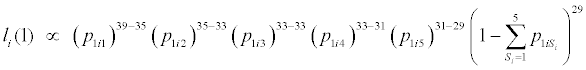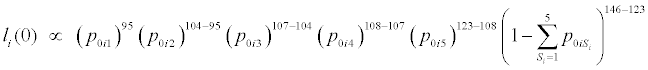One could also re-write li (1) and li (0) by expressing the probabilities in terms of the logit sensitivities and logit specificities , to directly estimate the logits and their variance-covariance matrix. Estimation of the likelihoods provides the within-study variances,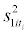and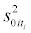, for the logit sensitivity and logit specificity estimates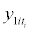and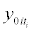, respectively, and crucially also the within-study covariance between logit sensitivity estimates for each pair of thresholds (e.g.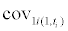is the covariance between y1i1 and), and similarly the within-study covariance between logit specificity estimates for each pair of thresholds (e.g.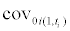is the covariance betweenand). As sensitivity and specificity are estimated on different sets of patients, there is always zero within-study correlation between each pair of logit sensitivity and logit specificity estimates at a given threshold.

If two or more thresholds give identical results for sensitivity (or specificity) in a study, it is not then possible to estimate both thresholds in the same likelihood. This can be seen in the above li(1) likelihood for the Al Ragib study, as p1i3 is not identifiable due to no diseased patients having a test value falling between the second and third thresholds; thus the observed sensitivity is the same at ti=2 and ti=3. In this situation only one of the thresholds can be included in the likelihood, and so in Al Ragib, for example, one can exclude threshold ti=3 and thus remove p1i3 in li(1). However the estimated logit value, its variance and covariances for the included threshold will be identical for the other excluded threshold(s). For this reason, the within-study correlation between thresholds with the same sensitivity (or specificity) will be 1, resulting in a singular covariance matrix. To make calculations possible, one can select the nearest-approximant positive definite matrix ; an easier to implement approach is to simply reduce the corrections slightly (e.g. map ± 1 to ± 0.95).

Step 2: Multivariate meta-analysis

The y1iti and y0iti obtained from step 1 for all thresholds can now be synthesised simultaneously in a multivariate meta-analysis that accounts for their within-study correlations. We now discuss two options for the multivariate model, one that treats the true logit sensitivities and logit specificities as separate (ordered) parameters, and one that assumes that they are monotonic functions of the (continuous) threshold.

Option (i) - Multivariate meta-analysis with separate parameters for test performance at each threshold

Let there be a total of t=1 to T different thresholds across all studies (e.g. T=23 for the PCR data in Table 1), and let these be ordered in increasing value. Then in each study the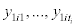and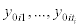can be linked to one of these thresholds. If all studies report all thresholds, a multivariate-normal meta-analysis model assumes that: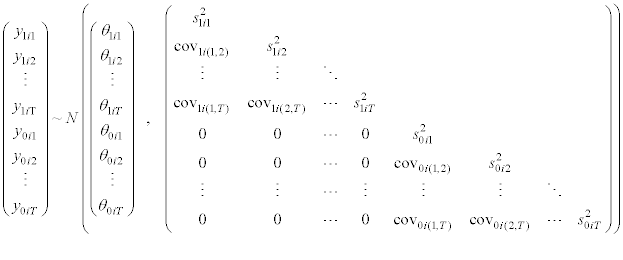where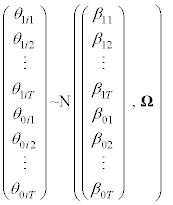(5)

We might add ordering constraints so that mean logit sensitivities (mean logit specificities) decrease (increase) as the thresholds become higher: that is to constrain θ1ij≥θ1ik, β1j≥β1k0ikθ0ij, β0k≥β0j for j<k.

In model (5) Ω is a 2T by 2T matrix representing the between-study variance-covariance matrix for the logit sensitivity and logit specificity values. The study estimates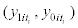, their variances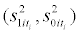, and covariances (e.g.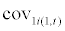) are available from step one for each study. The variances and covariances are assumed known, which is a common meta-analysis assumption . The model can easily accommodate missing thresholds in a study, assuming they are missing at random, just as described elsewhere for missing outcomes in a multivariate meta-analysis of multiple outcomes [11,27]. The model can be fitted using maximum likelihood estimation or restricted maximum likelihood (REML), for example in SAS Proc MIXED.

The β1t and β0t terms give the average logit sensitivity and average logit specificity at threshold t, respectively, and can be transformed back to give the summary sensitivity (exp (β1t)/(1+exp (β1t)) and the summary specificity ((exp (β0t)/(1+exp (β0t)).

The number of parameters in the model is quadratic in T: one has to estimate 2T means, 2T variances and T (2T-1) correlations. This makes fitting the model impractical for modest or large numbers of thresholds. For the PCR example (Table 1), this model would have 1127 parameters. Alternatively, one could impose structure to Ω, to reduce the number of parameters to be estimated. We do not explore alternative structural specifications in this work. An additional complication is that one has to use convex optimization techniques to maximize the likelihood of the model, while respecting the inequality constraints. For these reasons, model (5) will often be impractical.

Option (ii)-Multivariate meta-analysis assuming a functional relationship between test performance and threshold

One can drastically reduce the number of parameters in the previous model by assuming that the true logit sensitivity (logit specificity) is a monotonic decreasing (increasing) function of the threshold. The simplest form is a linear relationship such that a one-unit increase in threshold value, x, leads to a constant change in logit sensitivity of γ1, and a constant change in logit specificity of γ0, with these changes assumed to be fixed across studies: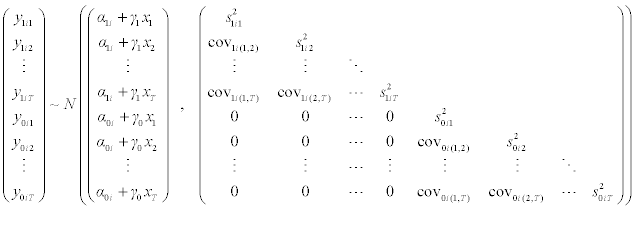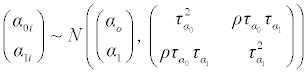(6)

where xt is the threshold value at the tth available threshold (where t=1 to T, and T is the total number of different thresholds considered across all studies), and model (6) is subject to the constraints γ1≤ 0, γ0 ≥ 0.

In model (6) α1 and α0 are average intercepts,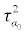and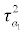τ are between-study variances and ρ the between-study correlation. Model (6) is a multivariate mixed effects (random intercept/fixed slope) meta-regression with threshold as the only covariate. The effect of threshold on logit sensitivity and logit specificity is allowed to differ through different intercepts (α1i and α0i) and slopes (γ1 and γ0). The summary ROC curve is therefore defined by α1, α0, γ1 and γ0. Model (6) has only 7 parameters instead of 1127 in model (5), at the cost of making strong assumptions about how test performance changes across thresholds. Extensions to model (6) may specify random slopes, but this may complicate estimation; indeed such an extension did not converge for the PCR example.

Fitting model (6) accounting for the constraints is straightforward (e.g. using REML in SAS Proc MIXED). As above one can accommodate missing thresholds in studies, assuming that they are missing at random, for example through a data-augmentation approach, where here the variances of missing threshold estimates are given a large value (e.g. 100000, and thus zero weighting) and zero covariances. Example SAS code for the data-augmentation and model fitting is available on request by the first author.

Summary estimates for logit sensitivity and specificity can be obtained after fitting model (6) for any threshold of choice (even those not reported in any of the studies) by:

logit sensitivity estimate at threshold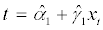(7)

logit specificity estimate at threshold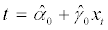(8)

These can be transformed back to the sensitivity and specificity scale, and plotted together to produce a summary ROC curve to aid examination of test accuracy. Note that each point on the summary ROC curve relates to a known threshold value; this is not true for summary ROC curves derived from meta-analyses using a single threshold result from each study .

The linear relationships assumed in model (6) are a common assumption in meta-analysis of test accuracy studies producing summary ROC curves . However other specifications can be examined, and the fit of the model investigated using statistics such as AIC and BIC. For example, below we extend model (6) to allow a cubic relationship: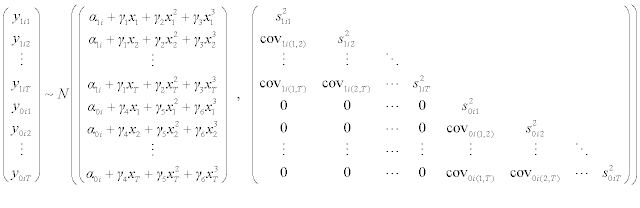(9)

where the model is subject to the constraint that the polynomial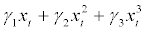is decreasing and the polynomial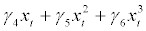is increasing in the range of interest for the threshold values xt. The optimization of the constrained likelihood for model (9) may be difficult for some datasets, depending on the proportion of missing thresholds in studies.

#### Results

We now apply the methods described in Section 3 to the PCR data introduced in Section 2. Findings are described for each step of the methods outlined.

Step 1: Individual study results

In the PCR data, there are 23 different thresholds across studies (T=23). For each study we used SAS Proc NLMIXED to specify and then maximise the log of likelihoods (1) and (2) , to obtain estimatesandfor each available threshold in that study, and their withinstudy covariance matrix. Within-study correlations were generally very high and largest for pairs of thresholds closer together, emphasising the rationale for analysing all thresholds simultaneously. For example, in the Al Ragib study the correlation matrix for the logit sensitivity estimates was as follows:

 Correlation matrix Threshold value,xt 0.13 0.18 0.19 0.2 0.49 0.13 1 0.7928 0.7928 0.6655 0.5757 0.18 0.7928 1.0000 1.0000 0.8394 0.7261 0.19 0.7928 1.0000 1.0000 0.8394 0.7261 0.2 0.6655 0.8394 0.8394 1.0000 0.8651 0.49 0.5757 0.7261 0.7261 0.8651 1.0000

Notice here that the correlation matrix is singular (there is a correlation of +1 between logit sensitivities for thresholds 0.18 and 0.19). As mentioned in the methods, to allow estimation of meta-analysis models one can truncate correlations larger than 0.95, to obtain an approximant invertible correlation matrix.

Step 2: Meta-analysis results

We transferred the y1it, y0it and within-study covariance matrix to SAS for all studies and fitted the multivariate-normal meta-analysis model (6) using REML. (Model (5) was not fit because for T=23 thresholds it has many more parameters than the available data points). The model converged after about one hour, and the parameter estimates are shown in Table 2.

Estimate Standard error
Intercepts
α0 0.851 0.354
α1 2.863 0.293
Slopes
γ0 2.309 0.307
γ1 -3.347 0.419
Variance-covariance terms1.3690.687
ρ 0.935

Table 2: REML estimates of parameters in model (6) when applied to the PCR data.

Summary ROC curve from model (6): The summary ROC curve from model (6) assumes a linear relationship between threshold value and logit sensitivity and logit specificity. The resultant curve in ROC space is shown in Figure 1, and the summary results for each threshold are shown in Table 3. Assuming that sensitivity and specificity are equally important (and thus that test accuracy is equally important for those with and without proteinuria), the threshold for which the sum of sensitivity and specificity is maximum is around a PCR value of 0.30 to 0.35. PCR thresholds between 0.24 and 0.40 give summary sensitivity and specificity values that are both above 80%, and a PCR value of 0.35 gives a similar summary sensitivity and specificity of 0.84.

Figure 1: Pair of summary sensitivity and specificity results for each threshold value, plotted in ROC space, for three different models: (i) model (6) with a linear trend, (ii) model (6) extended to include a quadratic trend, and (iii) model (6) with a cubic trend (i.e. model (9)).

Threshold value, x No. studies with this threshold Sensitivity Specificity
Summary estimate  95% CI Summary estimate 95% CI
lower  upper lower upper
0.13 1 0.919 0.869 0.951 0.760 0.615 0.862
0.14 2 0.916 0.865 0.949 0.764 0.620 0.865
0.15 5 0.914 0.862 0.947 0.768 0.626 0.868
0.16 3 0.911 0.858 0.946 0.772 0.631 0.870
0.17 3 0.908 0.854 0.944 0.776 0.637 0.873
0.18 3 0.906 0.850 0.942 0.780 0.642 0.875
0.19 4 0.903 0.846 0.940 0.784 0.648 0.878
0.2 6 0.900 0.842 0.938 0.788 0.653 0.880
0.21 3 0.897 0.837 0.936 0.792 0.658 0.883
0.22 1 0.893 0.833 0.934 0.796 0.663 0.885
0.23 1 0.890 0.828 0.932 0.799 0.668 0.887
0.24 2 0.887 0.824 0.929 0.803 0.673 0.890
0.25 2 0.884 0.819 0.927 0.807 0.679 0.892
0.28 2 0.873 0.804 0.920 0.817 0.693 0.898
0.3 4 0.865 0.793 0.915 0.824 0.703 0.903
0.31 1 0.861 0.788 0.912 0.827 0.708 0.905
0.32 1 0.857 0.782 0.909 0.831 0.712 0.907
0.35 1 0.844 0.764 0.901 0.840 0.726 0.912
0.39 2 0.826 0.739 0.888 0.852 0.743 0.920
0.4 2 0.821 0.732 0.885 0.855 0.748 0.922
0.45 1 0.795 0.697 0.868 0.869 0.768 0.930
0.49 1 0.773 0.666 0.853 0.879 0.783 0.936
0.5 2 0.767 0.658 0.849 0.881 0.787 0.937

Table 3: Summary results from REML estimation of meta-analysis model (6) (linear relationship).

However, it is important to note the large estimated variances of the intercept terms in model (6), signalling large between-study heterogeneity in test accuracy at each threshold (and thus large heterogeneity in the ROC curve itself). The between-study standard deviation in logit sensitivity and logit specificity is 0.83 and 1.17 respectively: this indicates that, for example at a threshold with a summary sensitivity and specificity of 80%, in a single population the true sensitivity may vary from 0.43 to 0.95 and the true specificity vary between 0.28 and 0.98, approximately. The heterogeneity may be attributable to between-study differences in the enrolled populations or in the methods of measuring PCR, and indicates that further research is essential to establish the causes of heterogeneity. This is beyond the aims of this paper, but model (6) can be extended to examine additional study-level covariates that may explain the heterogeneity, at least in part. Note there was also a large between-study correlation of 0.94 between the model intercepts for sensitivity and specificity (Table 2), emphasising that studies with a higher true sensitivity are likely to have a higher true specificity.

Summary ROC curve from non-linear extensions to model (6): As mentioned, model (6) can also be extended to allow non-linear relationships between threshold value and logit sensitivity and logit specificity. We considered quadratic and cubic extensions to model (6): the cubic extension is as written in model (9). The summary ROC curves are shown in Figure 1, and the cubic model was the better fit (AIC: 5548 for cubic, 5591 for quadratic, 5605 for linear). Other fractional polynomial terms were also considered, but did not improve model fit. The cubic model stretches out the summary ROC curve compared to linear and quadratic models, and in particular gives lower summary specificity estimates at the lower PCR thresholds. The summary results from the cubic model are shown in Table 4, and the fit of the cubic model is shown in Figure 2. As before, when assuming sensitivity and specificity are equally important, the best threshold appears to be around 0.30 to 0.35.

Figure 2: Fit of meta-analysis model (9) with a cubic summary relationship between threshold value and (a) the study logit sensitivity estimates, and (b) the study logit specificity estimates.

Threshold value, x No. studies with this threshold Sensitivity Specificity
Summary estimate 95% CI Summary estimate 95% CI
lower   upper lower   upper
0.13 1 0.941 0.896 0.967 0.698 0.544 0.818
0.14 2 0.936 0.889 0.963 0.720 0.572 0.832
0.15 5 0.930 0.882 0.959 0.740 0.597 0.845
0.16 3 0.924 0.875 0.955 0.756 0.618 0.856
0.17 3 0.919 0.867 0.951 0.770 0.637 0.865
0.18 3 0.913 0.859 0.948 0.782 0.653 0.873
0.19 4 0.907 0.851 0.944 0.793 0.666 0.880
0.2 6 0.902 0.843 0.940 0.801 0.678 0.885
0.21 3 0.896 0.835 0.937 0.809 0.688 0.890
0.22 1 0.891 0.827 0.933 0.815 0.697 0.894
0.23 1 0.886 0.820 0.930 0.820 0.704 0.897
0.24 2 0.881 0.812 0.926 0.824 0.709 0.900
0.25 2 0.876 0.805 0.923 0.828 0.714 0.902
0.28 2 0.862 0.786 0.914 0.834 0.724 0.907
0.3 4 0.854 0.774 0.909 0.837 0.727 0.908
0.31 1 0.850 0.768 0.906 0.838 0.729 0.909
0.32 1 0.846 0.763 0.904 0.839 0.730 0.909
0.35 1 0.835 0.748 0.897 0.841 0.733 0.910
0.39 2 0.822 0.729 0.888 0.846 0.740 0.914
0.4 2 0.819 0.724 0.886 0.848 0.743 0.915
0.45 1 0.800 0.699 0.874 0.867 0.770 0.927
0.49 1 0.781 0.674 0.860 0.892 0.806 0.943
0.5 2 0.775 0.665 0.857 0.900 0.816 0.948

Table 4: Summary results from REML estimation of meta-analysis model (9) (cubic relationship).

Comparison to a separate analysis at each threshold: When presented with multiple thresholds per study, researchers often do a separate meta-analysis for each threshold. In comparison to a multivariate meta-analysis, this loses information and may make summary results hard to interpret. For example, consider a PCR threshold of 0.23: this was only considered by one study ('Yamasmit', Table 1). Based on this single study, the summary result for sensitivity is 0.95. A threshold of 0.13 is also considered by just one study ('Al Ragib', Table 1), and the summary sensitivity is 0.90. Therefore the higher threshold of 0.23 is giving a higher sensitivity estimate; this is clearly not helpful, as the summary sensitivity should decrease as threshold increases. In contrast, the multivariate model (6) (and its extensions such as model (9)) constrain results, so that the summary sensitivity estimates are now more appropriate, being 0.92 and 0.89 for thresholds 0.13 and 0.23 respectively in model (6) (Table 3).

Summary results for entirely missing thresholds: Finally, model (6) and model (9) produce summary results for each threshold reported in the literature, but also allow summary results for other unreported thresholds of interest. For example, consider a PCR threshold value of 0.29. Results for this threshold were not reported in any of the studies, but following model (9) the summary logit sensitivity for the threshold can be obtained by 2 3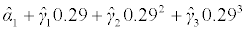and the summary specificity by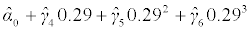. This gives a summary logit sensitivity of 0.858 and a summary specificity of 0.836, constrained to fall between the summary results for the reported thresholds of 0.28 and 0.30 (Table 4).

#### Discussion

Many meta-analysis articles have considered how to combine multiple test accuracy studies each presenting a single threshold, even if the threshold used in each study differs [1-8]. However, there have been only a few papers considering how to combine studies when some report results for multiple thresholds [13-15]. We have considered a multivariate-normal approach for this situation, and shown its potential benefits and limitations through an applied example. The approach allows all studies to be included, regardless of how many thresholds they present, and incorporates all reported thresholds even those just reported in a single study. The example shows that model (6) and subsequent extensions produce the more coherent results, constrained to be ordered across thresholds. It produces summary estimates of sensitivity and specificity for each threshold; estimates of between-study heterogeneity; and an admissible summary ROC curve, whilst always accounting for within-study correlation of results for multiple thresholds from the same study. As a consequence, the methods utilise more information than an approach that analyses each threshold independently, and thereby it reduces the impact of missing thresholds in the studies available. Summary results are produced for each threshold reported in the literature, and can even be derived for unreported thresholds that are of interest.

We note that our models (6) and (9) imply a specific distribution for test results in people with and without the condition of interest in each study. The same is true for any model that posits functionals between the true sensitivities (specificities) and the threshold (e.g., Hamza et al. , or Putter et al.). If the study-level data do not fit the implied distribution, predictions of summary sensitivity and specificity at various thresholds would be biased. Model (5) does not posit such functionals, and only assumes that true (logit) sensitivities and specificities at adjacent thresholds are ordered. However, relaxing the assumption comes at a large cost: for T=23 thresholds, model (5) has 1127 parameters, instead of only 7 in model (6). In situations with a smaller set of thresholds, for example T=2, 3 or 4, model (5) may be more feasible. Indeed, it may be preferable as with only a few thresholds models (6) and (9) may not converge: the fewer the data points the harder it is to estimate a functional relationship between the true sensitivities (specificities) and the threshold. In situation with only a few studies none of models (5), (6) or (9) maybe achievable, especially when the number of thresholds is large. The issue would be resolved if individual participant data (IPD) were available for each study, as then all thresholds are available for all studies. This allows the functional relationship to be estimated separately in each study based on the discrete (exact) likelihood , and the parameter estimates of the function then pooled across studies, similar to the IPD meta-analysis of longitudinal data .

Multivariate meta-analysis models are increasingly used to synthesise multiple correlated outcomes in meta-analysis , to improve the efficiency of summary estimates by borrowing strength across outcomes and thereby reducing the impact of missing data [12,36]. In this work we used the multivariate normal approximation to the multinomial distribution to model the observed data in each study. This approximation may be unsatisfactory for small sample sizes or for proportions near 0 or 1 [2,37,38]. In many applied examples, this limitation may not be a big problem. The advantage is that it is very easy to fit within standard software. Alternative models use discrete likelihoods for the observed data (e.g. see Hamza et al. ). However, they are more difficult to fit with random-effects and missing data, and often they do not converge . One could simplify matters by meta-analysing each threshold separately and excluding studies that do not report that threshold: this would allow the exact binomial distribution and removes studies with missing data . However, taking each threshold separately does not ensure summary results are ordered across thresholds, especially if studies have missing thresholds as in the PCR data. Therefore, although approximate, the proposed multivariatenormal model remains appealing, and may be considered in place of models using discrete likelihoods, for example when they fail to converge (as they did for the PCR data). We assumed the logit transformed sensitivity and specificity estimates follow a multivariate normal distribution in each study, but note log-log or probit transformations could also be considered if more suitable .

Our methods also allow studies providing IPD to be combined with studies only providing two by two tables for a partial set of thresholds. Essentially two by two tables for a full set of thresholds can be derived in IPD studies, which can then be combined with the other studies using the methods proposed. IPD would also allow the distribution of continuous test values for diseased and non-diseased patients to be modelled in each study; these distribution might then be synthesised across studies to form summary distributions, from which the summary ROC could easily be derived. With only aggregate data from non-IPD studies it is harder to derive the distributions of the original data, but they might be approximately calculable and we are considering this in further research.

In some situations sensitivity analyses may be useful, where the multivariate model is re-fitted to a subset of available studies. For example, if some studies are classed at a high risk of bias then one might investigate if summary meta-analysis conclusions are sensitive to their inclusion. Similarly if studies that use a different reference standard or method of test measurement may be omitted for sensitivity analysis. This was not considered in the original PCR meta-analysis , and so was not evaluated here.

Finally, we note that model (6) can be extended to compare multiple tests. Additional covariates can be included in the regression part of the model to distinguish each test, and if some studies consider two or more tests, the within-study correlation in the multiple test results can be accounted for in the same manner as described. The shape of the summary ROC curve may be forced to be the same for each test, or allowed to differ. Either way, one can compare the summary ROC curves obtained for the tests, to determine which test is more accurate .

#### Conclusion

We have presented a multivariate-normal method to deal with multiple and missing thresholds across studies in a meta-analysis. The method offers an approximate alternative when more exact methods experience computational problems or require complete data. By utilising correlation between thresholds the method limits the impact of missing threshold results from primary studies and ensures a summary ROC curve that, unlike a separate meta-analysis at each threshold, is constrained to be ordered across thresholds.

#### Acknowledgements

RDR and JJD were supported by funding from an MRC Methodology Research Grant in Multivariate Meta-analysis (grant reference number: MR/J013595/1). RKM is funded by an NIHR Clinical Lectureship.

#### References

Select your language of interest to view the total content in your interested language

### Article Usage

• Total views: 12617
• [From(publication date):
June-2014 - Jul 19, 2019]
• Breakdown by view type
• HTML page views : 8779Can't read the image? click here to refresh